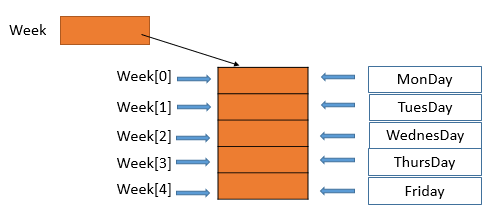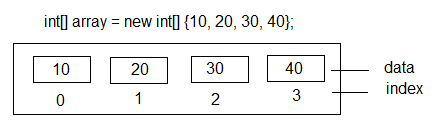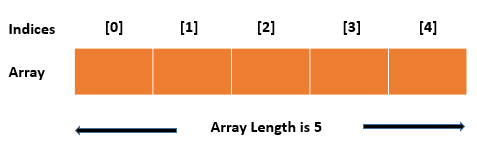C# Tutorial

# C# Array Examples

An array is a collection of data items that share a common name and data type. It is a fundamental data structure in computer programming, and it provides a convenient way to store and manipulate a large number of values of the same type. In C#, arrays are objects, which means they have properties and methods that can be used to manipulate their contents.

## String Array and integer Array

A string array is an array of string elements, and an integer array is an array of integer elements. You can create, initialize, and manipulate String Array and integer Array using C#. Also, you can perform various operations on both string and integer arrays, such as iterating through the elements, sorting the elements, or searching for a specific element. To perform these operations, you can use built-in methods provided by C#, such as Length, ForEach, Sort, IndexOf etc.

## C# String ArrayC# string array is an array of string elements, where each element is a sequence of characters. To create a string array in C#, you can use the following syntax:

string[] cArray = new string;

In the example above, declare a string array with three elements. To initialize the elements of the array, you can assign a value to each element as follows:

cArray = "Red"; cArray = "Blue"; cArray = "Green";

You can also create a string array with initialized elements as follows:

string[] cArray = new string[] { "Red", "Blue", "Green" };

## C# integer ArrayC# integer array is an array of integer elements, where each element is a whole number. To create an integer array in C#, you can use the following syntax:

int[] cArray = new int;

In the above example, declare an integer array with three elements. To initialize the elements of the array, you can assign a value to each element as follows:

cArray = 100; cArray = 200; cArray = 300;

You can also create an integer array with initialized elements as follows:

int[] cArray = new int[] { 100, 200, 300 };

## Accessing C# Array Elements

Once an array is created, its elements can be accessed using an index. In C#, array indexes start at zero, which means the first element is at index 0, the second element is at index 1, and so on. The following code demonstrates how to access the elements of an array:

int[] cArray = new int; cArray = 100; cArray = 200; int sum = cArray + cArray; Console.WriteLine(sum); //Output: 300

In the example above, the first line assigns the value 100 to the first element of the array, the second line assigns the value 100 to the second element, and the third line adds the values of the first two elements and assigns the result to the variable sum.

Similarly you can access the String array elements using index.

string[] cArray = new string; cArray = "Red"; cArray = "Blue"; cArray = "Green"; Console.WriteLine(cArray); //Output: Blue

## Looping Through C# Array

One common use of arrays is to loop through their elements and perform some operation on each element. C# provides several ways to loop through an array, including the for loop, the foreach loop etc.

### C# array using foreach Loop

In C#, you can use a foreach loop to iterate through the elements of an array. The foreach loop is a simplified way to iterate through the elements of an array, and is often used when you only need to read the values of the elements and don't need to access their indexes.

Following is an example of how to use a foreach loop to iterate through the elements of an integer array:

int[] cArray = new int[] { 100, 200, 300 }; foreach (int element in cArray) { Console.WriteLine(element); }
//Output: 100 200 300

The code above creates an integer array with three elements and assigns it to the variable cArray. The foreach loop is then used to iterate through each element of the array, and print its value to the console. In each iteration of the loop, the current element of the array is assigned to the variable element.

Similarly, you can use a foreach loop to iterate through the elements of a string array:

string[] cArray = new string[] { "Red", "Blue", "Green" }; foreach (string element in cArray) { Console.WriteLine(element); }
//Output: Red Blue Green

In the above code, the foreach loop is used to iterate through each element of the string array and print its value to the console.

The foreach loop can be useful when you only need to read the values of the elements and don't need to access their indexes. However, if you need to access the index of each element, or modify the elements of the array, you should use a for loop instead.

### C# array using for Loop

The following code demonstrates how to use a for loop to iterate through the elements of an array:

string[] cArray = new string[] { "Red", "Blue", "Green" }; for (int i = 0; i < cArray.Length; i++) { Console.WriteLine("Element {0} = {1}", i, cArray[i]); }
//Output: Element 0 = Red Element 1 = Blue Element 2 = Green

In the example above, the for loop starts at index 0 and continues until it reaches the end of the array, which is indicated by the Length property of the array. The code inside the loop prints the index and value of each element.

## How to find length of C# arrayIn C#, you can find the length of an array using the Length property. The Length property returns the number of elements in the array.

Following is an example of how to find the length of an integer array:

int[] cArray = new int[] { 100, 200, 300 }; int length = cArray.Length; Console.WriteLine("The length of the array is " + length); //Output: The length of the array is 3

The code above creates an integer array with three elements and assigns it to the variable cArray. The Length property is then used to get the length of the array, which is 3. The length is assigned to the variable length, and is printed to the console using Console.WriteLine.

Similarly, you can find the length of a string array:

string[] cArray = new string[] { "Red", "Blue", "Green" }; int length = cArray.Length; Console.WriteLine("The length of the array is " + length); //Output: The length of the array is 3

## Searching an item in C# Array

You can use the System.Array class methods such as Array.IndexOf() to find the index of an item in an array. For example, the following code searches for the item "Blue" in a string array using the Array.IndexOf() method:

string[] cArray = new string[] { "Red", "Blue", "Green" }; string itemToSearch = "Blue"; int index = Array.IndexOf(cArray, itemToSearch); if (index >= 0) { Console.WriteLine("Item found at index " + index); } else { Console.WriteLine("Item not found"); } //Output: Item found at index 1

The Array.IndexOf() method returns the index of the first occurrence of the specified item in the array, or -1 if the item is not found. In this example, the index of the item "Blue" is printed to the console.

### Using for loop

You can also search for an item in an array using a loop or using built-in methods provided by the System.Array class.

Here is an example of how to search for an item in an integer array using a loop:

int[] cArray = new int[] { 100, 200, 300 }; int itemToSearch = 200; bool found = false; for (int i = 0; i < cArray.Length; i++) { if (cArray[i] == itemToSearch) { found = true; Console.WriteLine("Item found at index " + i); break; } } if (!found) { Console.WriteLine("Item not found"); } //Output: Item found at index 1

The code above creates an integer array with three elements and assigns it to the variable cArray. The item to search for is assigned to the variable itemToSearch. A flag variable found is initialized to false.

## How to sort C# array

In C#, you can sort an array in ascending or descending order using built-in methods provided by the System.Array class.

Here is an example of how to sort an integer array in ascending order:

int[] cArray = new int[] { 300, 100, 200 }; Array.Sort(cArray); foreach (int element in cArray) { Console.WriteLine(element); }
//Output: 100 200 300

The code above creates an integer array with three elements and assigns it to the variable cArray. The Array.Sort() method is then used to sort the array in ascending order.

The foreach loop is used to iterate through each element of the sorted array and print its value to the console.

Similarly, you can sort a string array in ascending order:

string[] cArray = new string[] { "Red", "Blue", "Green" }; Array.Sort(cArray); foreach (string element in cArray) { Console.WriteLine(element); }
//Output: Blue Green Red

In the above case, the Array.Sort() method is used to sort the string array in ascending order.

### Array.Reverse();

To sort an array in descending order, you can use the Array.Reverse() method after sorting the array in ascending order. Here is an example of how to sort an integer array in descending order:

int[] cArray = new int[] { 200, 300, 100 }; Array.Sort(cArray); Array.Reverse(cArray); foreach (int element in cArray) { Console.WriteLine(element); }
//Output: 300 200 100

In the above example, the Array.Sort() method is used to sort the integer array in ascending order. The Array.Reverse() method is then used to reverse the order of the sorted array, resulting in a descending order.

## C# array Passing as Argument

In C#, you can pass an array as an argument to a method. This allows you to manipulate the contents of the array within the method, and any changes made to the array are reflected in the original array outside of the method.

Here is an example of how to pass an integer array as an argument to a method:

static void DoubleArrayValues(int[] cArray) { for (int i = 0; i < cArray.Length; i++) { cArray[i] *= 2; } } static void Main(string[] args) { int[] cArray = new int[] { 100, 200, 300 }; DoubleArrayValues(cArray); foreach (int element in cArray) { Console.WriteLine(element); } }
//Output: 200 400 600

In the above example, the DoubleArrayValues method takes an integer array cArray as an argument. The method iterates through the array using a for loop and

## C# Multi-Dimensional Array

C# also supports multi-dimensional arrays, which are arrays that have more than one dimension. Multi-dimensional arrays can be declared and initialized in a similar way to single-dimensional arrays, except that you specify the number of elements for each dimension. The following code demonstrates how to create a two-dimensional array:

int[,] cArray = new int[3, 4];

In the example above, the array has three rows and four columns.

## C# Jagged Arrays

Another type of array that C# supports is the jagged array, which is an array of arrays. Jagged arrays can have different lengths for each element, and they are created by initializing each element of the array with its own array. The following code demonstrates how to create a jagged array:

int[][] myArray = new int[]; myArray = new int { 1, 2, 3, 4 }; myArray = new int { 5, 6 }; myArray = new int { 7, 8, 9 };

In the example above, the jagged array has three elements, each of which is an array with a different number of elements.

## Conclusion:

The study of C# arrays involves understanding how to create, access, and manipulate arrays, as well as how to use loops to iterate through their elements. Additionally, it involves understanding the different types of arrays that C# supports, including multi-dimensional arrays and jagged.